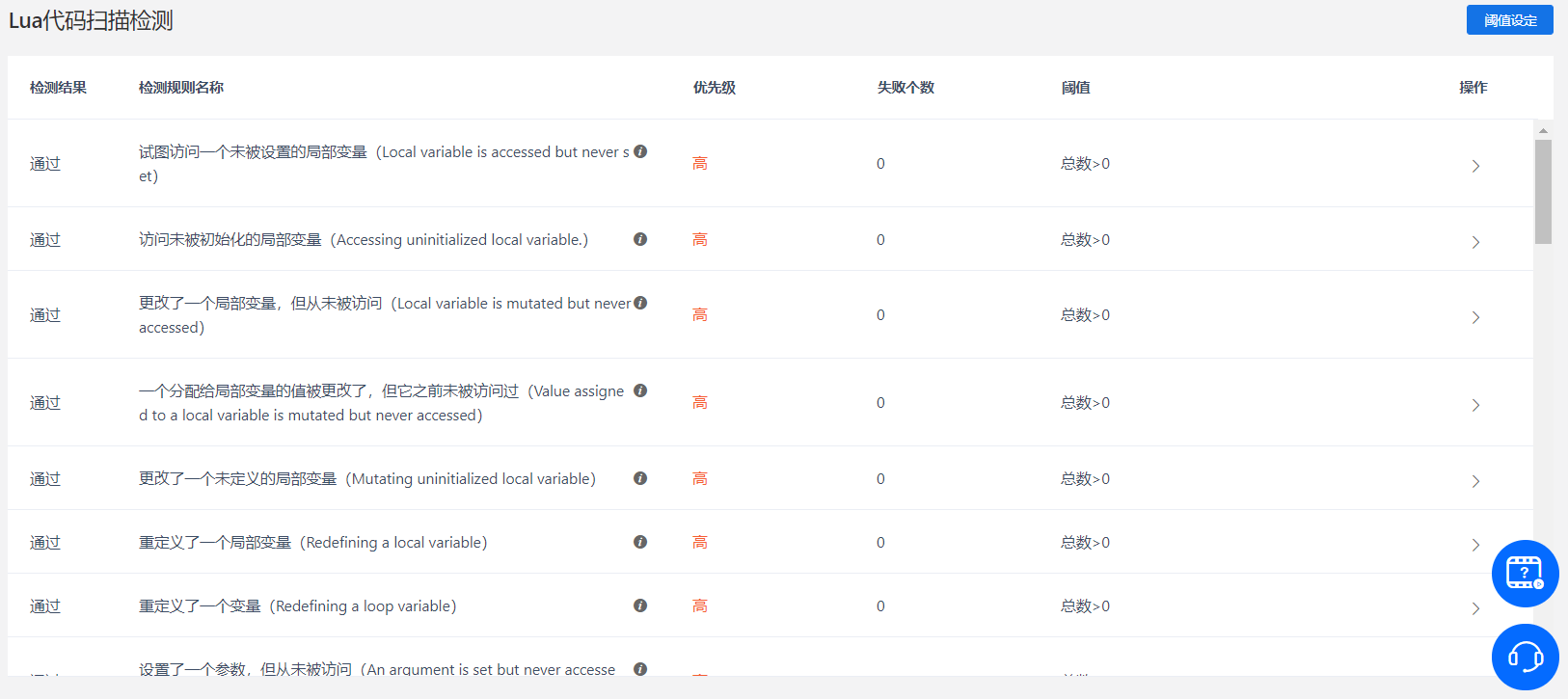# Lua优化——认识局部变量中的常见陷阱

### 1、局部变量相关

1.1 未被使用的局部变量``````local bar=5
``````

1.2 未被使用的参数变量``````function fun(x,y)
print(x)
end
``````

1.3 未被使用的循环变量``````for i=1,100 do
print("huh")
end
``````

1.4 访问了一个未被设置的局部变量``````local num
print(num.string)
``````

1.5 设置了一个局部变量，但从未被访问``````local num=5
num=6
``````

1.6 设置了一个参数变量，但从未被访问``````function fun(num)
num=6
end
``````

1.7 设置了一个循环变量，但从未被访问``````for i=1,100 do
i=2
end
``````

1.8 更改了一个局部变量，但从未被访问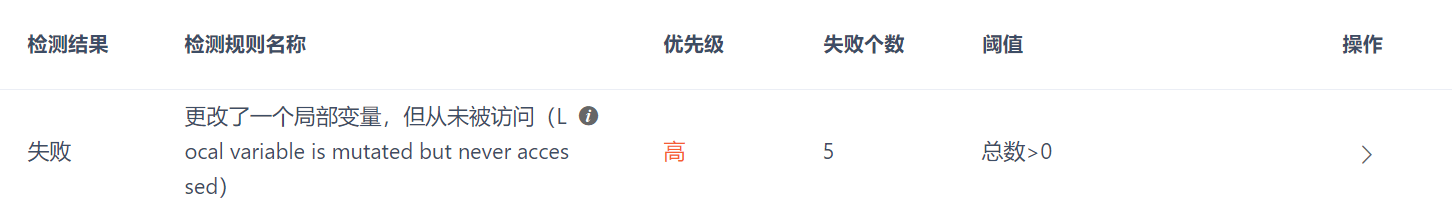``````local bar={"aaa"}
bar.dd='ff'
``````

### 2、值相关

2.1 未被使用的一个局部变量的值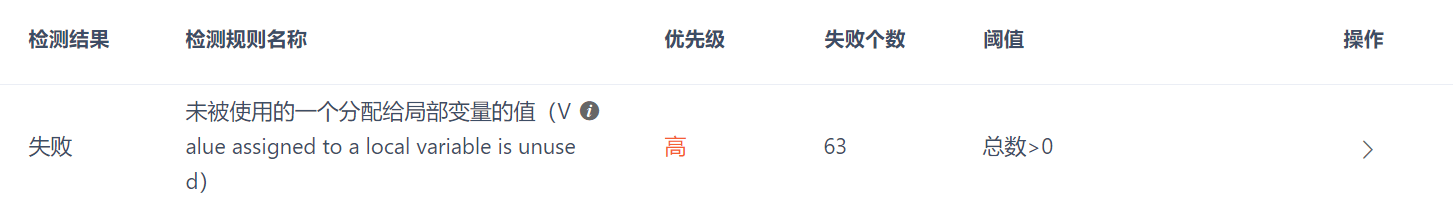``````local o = 5
print(o)
o=6
``````

2.2 未被使用的一个参数变量的值``````function fun(x)
x=3
print(x)
end
``````

2.3 未被使用的一个循环变量的值``````for index=1,100 do
index=2
print(index)
end
``````

2.4 未被使用的一个表文字域的值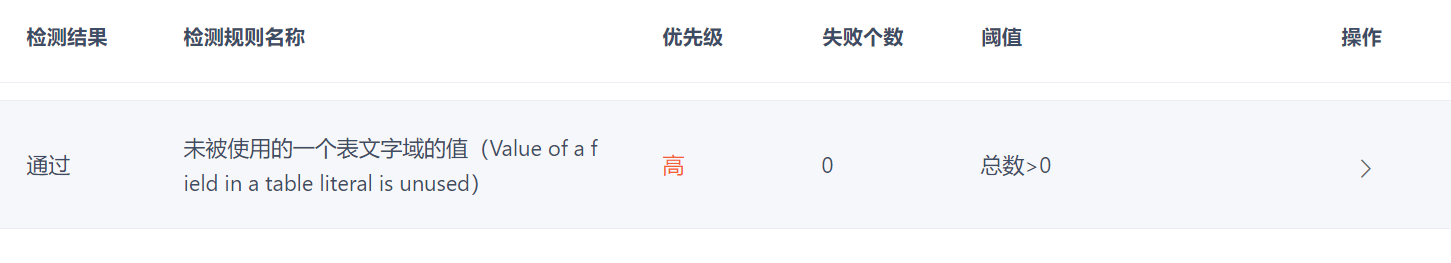``````local bar = {b = 1, b = 2}
``````

2.5 访问未被初始化的局部变量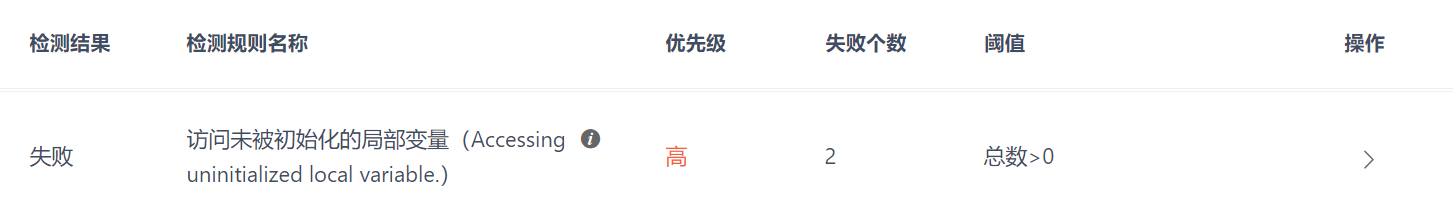``````local bar
if condition()
bar={}
else
print(bar)
``````

else分支语句中，访问了bar这个未初始化的局部变量。

2.6 更改了一个局部变量的值，但从未被访问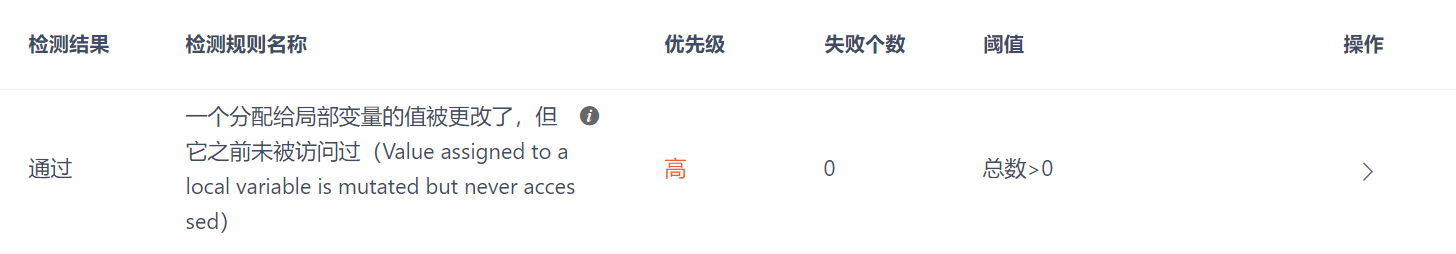``````local function fun(st)
if not st then
st = {}
end
st.aa=2
end

local num
fun(num)
print(num)
>nil
``````

2.7 更改了一个未被初始化的局部变量``````local bar
bar.a=2
bar={}
``````

### 3、与覆盖行为相关

3.1 重定义了一个局部变量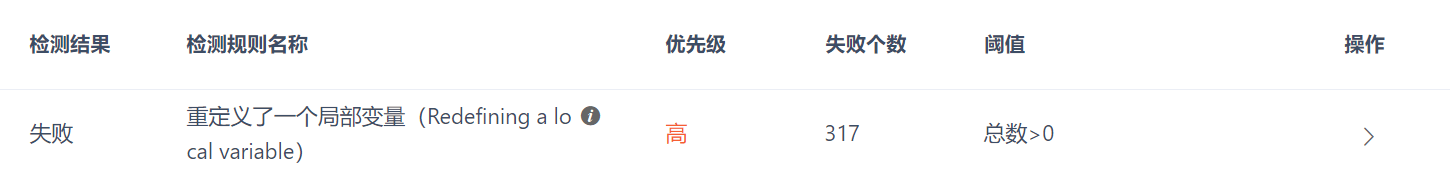``````local bar={}
local bar={"huh"}
``````

3.2 重定义了一个参数变量``````function fun(bar)
local bar={}
end
``````

3.3 重定义了一个循环变量``````for i=1,100 do
local i={}
end
``````

3.4 覆盖了一个局部变量``````local bar
for i=1,100 do
local bar={}
end
``````

3.5 覆盖了一个参数变量``````function fun(x)
if condition() then
local x={}
print(x)
else
print(x)
end
end
``````

3.6 覆盖了一个循环变量``````for i=1,100 do
if condition() then
local i={"huh"}
print(i)
else
print(i)
end
``````

3.7 覆盖了一个上值``````local bar
function fun()
local bar={}
end
``````

3.8 覆盖了一个上值参数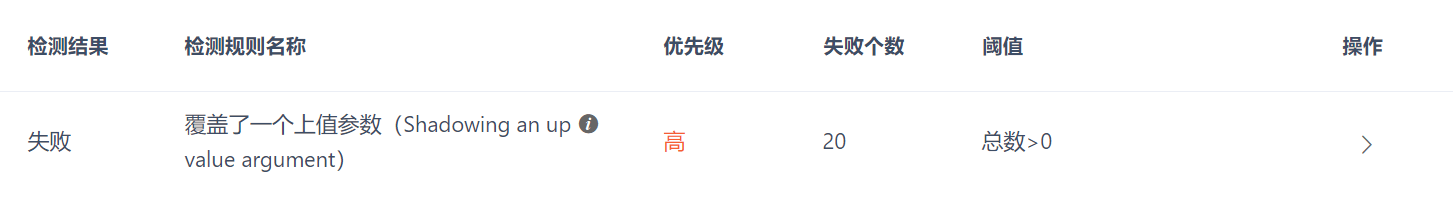``````function fun(bar)
local function fun2()
local bar={}
end
return fun2
end
``````

3.9 覆盖了一个上值循环变量``````for index=1,100 do
local function fun()
local index={}
end
end
``````